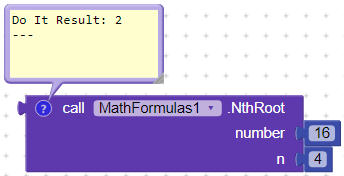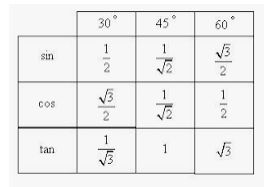# [Extension] Math Formulas [V3]

Thank you @ImranTariq!

2 Likes

1 Like

Thank you for liking @techVsurya!

1 Like

Great work​1 Like

# Changelog V2

### Added 6 blocks for calculating volumes of following:

1. Cube
2. Parallelepiped
3. Regular prism
4. Cylinder
5. Cone
6. Sphere

Check 1st post for the link.

# Changelog V3

1. Pi (Returns value of Pi)
2. Cube (Returns Cube of a number)
3. CubeRoot (Returns cube root of a number)
4. Log (Returns the natural logarithm of a number)
5. Log10 (Returns the base 10 logarithm of a number)
6. Log1p (Returns the natural logarithm of the sum of the argument and 1)
7. Exp (Returns E raised to the power of a number, where E is Euler’s number and it is approximately equal to 2.71828
8. Expm1 (Calculate the power of E and subtract one from it)
9. PowerOfNumber (Returns the power of a number)
10. QuadraticFormula (Find the value of Quadratic formula by providing values of a, b and c. Positive value will be returned)

Suggest me what should be added in next version?

2 Likes

Great extension! I suggest to add the n th root. It isn’t too hard. For example, 3√x = x^(1/3). Are you able to power using non whole exponents?

1 Like

a math extension is a good start into creating extensions…

are you aware, that there already is a Math extension, which is able to calculate “everything”?
and it uses only one method to do that…Taifun

6 Likes

Thank you @Robert!

Will try to add.Yeah! That’s why I started with it. I had no prior knowledge of creating extensions.

Sir, I know about every single extension of yours.
This page opens the whole time I’m using my PC or laptop:
App Inventor Extensions | Pura Vida Apps
You are great!You are my inspiration!

Yes I know, you can make anything simple. I’m just using `Java's Math class` for my experience.

1 Like

## Small Update### Usage ExampleP.S: Thank you @Robert for the idea!

1 Like

Thank you.

1 Like

You’re welcome.
Any other idea?
I would like to hear.

Well, you have done such a great job! This extension has a lot of blocks already. Currently I do not have other ideas.

2 Likes

mathematical functions are interesting of course.
but

• to make the functions work with the hexadecimal transformation ? for example, working with bytes … 04 6C 01 08 … as a result it is 01086C04
or 17 329 156. (the difficulty in assembling it is to control the number greater than 10 or not) .
• base for translation (basis) of representation of number from hexadecimal system in decimal at observance of base of numerical values (16-bit, 32,64)?
• Translation of numeric values into alphabetic values in both directions . For example, code 31=number 1, https://upload.wikimedia.org/wikipedia/commons/thumb/4/4f/ASCII_Code_Chart.svg/369px-ASCII_Code_Chart.svg.png

Well, thank you for your interest @11119!

You can clearly see from the name of extension that it is related to mathematical formulas (formulae).

Why do you think that your proposal is related to this extension?

Maybe I’m wrong.I would like to do a scientific account, but can it be done?

sin or cos or tan 30

The resultWhy you don’t use built-in math blocks?

https://docs.kodular.io/blocks/math/#sin

https://docs.kodular.io/blocks/math/#cos

https://docs.kodular.io/blocks/math/#tan

1 Like

I mean fractional view